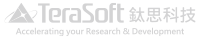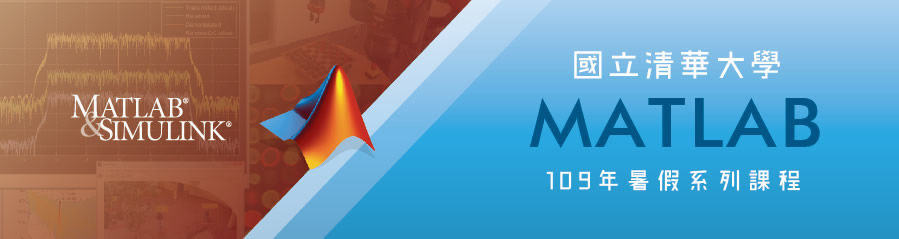## 【109年暑假MATLAB系列課程】

8/10中午12:00開放報名上課地點：校本部計通中心電腦教室 《Venue》CCC – Computer Laboratory 聯絡窗口： inquiry@cc.nthu.edu.tw 校內分機31231 江小姐Any inquiries, please mail to the following address: inquiry@cc.nthu.edu.tw or call Miss Chiang at campus ext.31231

MATLAB 基礎程式設計 / MATLAB Fundamental

Date: 08/25 ; Time : 09:30~12:00; 13:00~16:30

• MATLAB操作介面介紹
• MATLAB繪圖工具之使用說明
• MATLAB各類變數型態之介紹與說明
• MATLAB之程式設計流程簡介

MATLAB 進階程式設計 / MATLAB Advance

Date: 08/26 ; Time : 09:30~12:00; 13:00~16:30

• 如何產生及呼叫MATLAB Function.
• 如何建構MATLAB Function Code，介紹各種不同類型的Function Type.
• 如何撰寫含有防呆機制的程式
• 如何針對程式碼進行除錯及如何提升程式碼的執行效率

MATLAB於影像處理的應用 / MATLAB for Image Processing

Date: 08/27 ; Time : 13:00~16:30

• Why should you use MATLAB for Image Processing?
• Images in MATLAB
• Image Enhancement
• Image Registration
• Image Analysis
• Image Segmentation

MATLAB於電腦視覺之應用 / MATLAB for Computer Vision

Date: 08/28 ; Time : 13:00~16:30

• Streaming Processing (System Object)
• Featured-Based Workflow
• Image Category Classification
• Object Detection and Stereo Vision

Simulink基礎技巧 / Simulink Basic

Date: 09/08 ; Time : 13:00~16:30

• Introduction
• Building the Model
• Creating A Simple Model
• Working with Subsystems
• Working with MATLAB
• Modeling Discrete Systems
• Modeling Continuous and Hybrid Systems